# Turtle Graphics  on Basic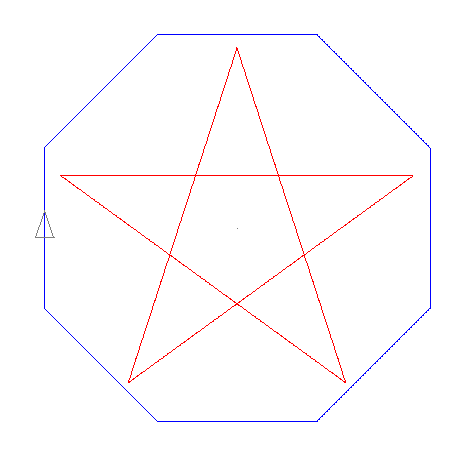Turtle graphics is the special drawing functions
it prepared for the LOGO language.
It was used for artificial intelligence and educational use.

The cursor like a turtle on the screen,
advance and rotational directions are given,
and the orbit will draw a pattern.

In the turtle graphics realized by a program of 'Basic',
plural tortoises can be moved at the same time by multi-turtle.

Usage example:

forward 80
right 30

80 move forward and rotate 30 degrees on the right.

It can be used from both command and program list.

File: "turtle.bas"
Version 2013/07/04 or later is required

Command list
The abbreviated form of two characters(Notation[ ]) is usable, too.

[fd]  forward n - n Move forward.
[bk]  back n - n Move back.
[rt]  right n - Right turn n.
[lt]  left n - Left turn n.
[wt]  wait n - It stop between n.
[pd]  pendown - Pen is lowered.
[pu]  penup - Pen is raised. The orbital trace isn't drawn.
[rp]  repeat n [ ] - The inside of brackets is repeated n times and performed.
Multiple structure is possible.
[cs]  cls - Clear screen.
[tc]  color n - Color n is chosen.(0~15). Same as Basic's coloration-col(n).
[pn]  pen n - In multiple cursors, No.n cursor is chosen.
[ps]  pos x y - It move to coordinates(x, y). Cann't change by inside of loop.
[mv]  move x y - Only x and y move from current position.
[ag]  angle n - It set to angle n.
[sp]  speed n - It set to speed n. (1~10). larger-faster(default:10)

Variable
It can be used from @0 to @255.
@v n    The value of formula n is assigned to the variable No.v.

Label & Jump
*label    To show a label name in the alphabet after '*'.

Conditional branch
if n    if the formula n is except '0', the next command is executed.
if it is '0', it will skip.

The parameter can use the same formula & functions as 'Basic'.
Surplus-'mod', so it need a blank for right and left,
it substitute with '%'.
Variable '@v' can also be used into a formula.

Three functions for exclusive use of TG are usable, too.
posx(n)    To get the coordinate X of pen n.
posy(n)    To get the coordinate Y of pen n.
angle(n)    To get the angle of pen n.

The command only for direct
It isn't necessary to enclose the file name by "".
[md]  mode n    Drawing mode change.
0: It draw with the cursor move animation.
1: It draw in straight without animation.

The coordinate origin(0,0) is center of screen,
the right and upper is Plus, the left and under is Minus.

Upper direction is angle of 0 degrees,
It is clockwise and grow big.(0~359)

Usage example:

Variable
@1 100 @2 posx(2) @1 sqr(@1+@2)
@1=100, @2=pen2:x, @1=Square root (@1+@2)

Label
fd 20 *point1 rt 30 fd 40 go point1
forward20, right30, forward40, and goback to front of rt 30.

Branch
if angle(1)>180 lt 2 fd 10
if angle:pen1 larger than 180, left turn2 and forward10.
if not, skip leftturn and next command-forward10.

Multi-turtle    Initial pen is No.1, and coordinates start in (0, 0).
tc 1 rt 90 fd 100 pn 2 tc 2 lt 90 sd 5 fd 100
Blue line run to the right, Red line run to the left at speed of half.

The pen can be simultaneously used to a maximum of 16.
When a value in (pen & color) is protruded more than the maximum,
it is reduced to the surplus value automatically.

The commands can be described to a text file and can also be executed from the 'load' command.
It divide by space, between commands, between parameters.

e.g.
The program of star and polygon. (page top)

file " tg0.lgo"
pn 1 tc 10 mv 0 180 ag 162 rp 5[fd 352 rt 144]
pn 2 tc 9 mv -193 80 ag 45 rp 8[fd 160 rt 45]

It can also edit and save by the editor of Basic for Android.
When line number is attached, it is removed and loaded.

10 pn 1 tc 10 mv 0 180 ag 162 rp 5[fd 352 rt 144]
20 pn 2 tc 9 mv -193 80 ag 45 rp 8[fd 160 rt 45]

When carrying out recursive processing,
it writes in directly among 100~999 lines of "turtle.bas."
The user function 'func endfunc' is used.
It is a portion of 100~250 lines of the next program.
Since line40 have 'input' for a command input, it must be removed by '(rem).
The portion of ' f=tgcom("left 60") ' is the function which send the command of a turtle.
It doesn't proceed to the next command until drawing command finishes.
When use variable of 'Basic' directly; like ' f=tgcom("forward "+str\$(ln)) ',
it connect with command-string using a function 'str\$()'.
It can setup drawing-mode by line30. (What requires time is set to mode=1)

File [ tg01.bas ]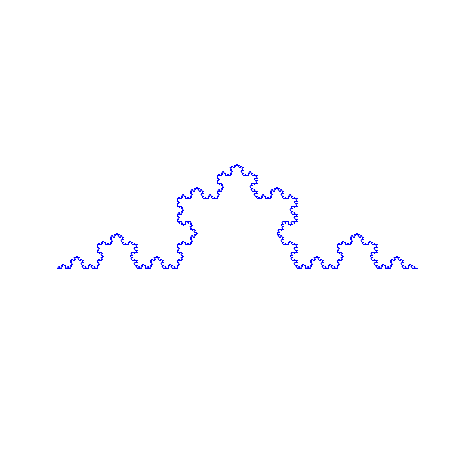10 'Turtle Graphics 1.00
20 gosub turtle: :f=tgini()
30d\$="":mode=1
40 'if d\$="" then input d\$:f=tgcom(d\$):goto 40
50 '
100 f=tgcom("angle 90 move -180 -40 tc 9")
110 f=koch(360)
120 '
130 func koch(ln)
140 if ln>4 then
150  f=koch(ln/3)
160  f=tgcom("left 60")
170  f=koch(ln/3)
180  f=tgcom("right 120")
190  f=koch(ln/3)
200  f=tgcom("left 60")
210  f=koch(ln/3)
220 else
230  f=tgcom("forward "+str\$(ln))
240 endif
250 endfunc
1000 '
1010 end
1020 turtle:
1030cls3:d0=0:tpen=1:f2=0:tmax=0:iflg=0:cnt=0:mode=0
1040 dim tx(16),ty(16),ta(16),tp(16),loopc(16,16),loopp(16,16)
1050 dim tmx(16),tmy(16),tc(16),taa(16),tac(16),lplv(16)
1060 ........

Sample
File [ tg04.bas ]
Load turtle.bas and run, and enter  [ load  tg04.bas ] then drawn.

rp 4096[@2 @2+1 @1 @1+@2 @3 @1/1000 @1 (@3-int(@3))*1000 @4 @4+1 tc @4 fd int(@3) rt 1]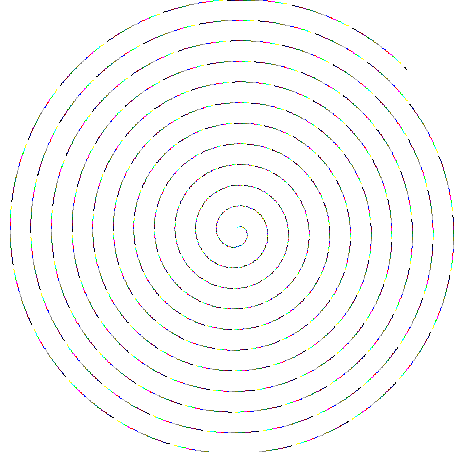File [ tg06.bas ]
Load turtle.bas and run, and enter  [ load  tg06.bas ] then drawn.

tc 5 @2 1 mv -50 -50 rp 30[@2 @2+1 tc @2 rp 3[fd 100+@1*6 rt 91]@1 @1+1]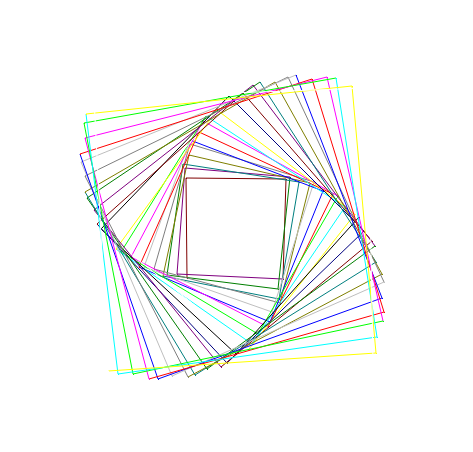File [ tg02.bas ]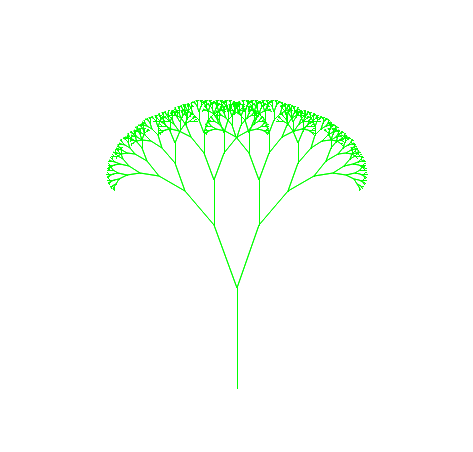30d\$="":mode=1
40 'if d\$="" then input d\$:f=tgcom(d\$):goto 40
50 '
100 r=0.6666666666666666
110 ang=20
120 ln2=2
130 f=tgcom("move 0 -160 tc 12")
140 '
150 f=branch(100)
160 '
170 func branch(ln)
180  if ln<=ln2 then endfunc
190  f=tgcom("forward "+str\$(ln))
200  f=tgcom("left "+str\$(ang))
210  f=branch(r*ln)
220  f=tgcom("right "+str\$(2*ang))
230  f=branch(r*ln)
240  f=tgcom("left "+str\$(ang))
250  f=tgcom("penup back "+str\$(ln)+" pendown")
260 endfunc

File [ tg03.bas ]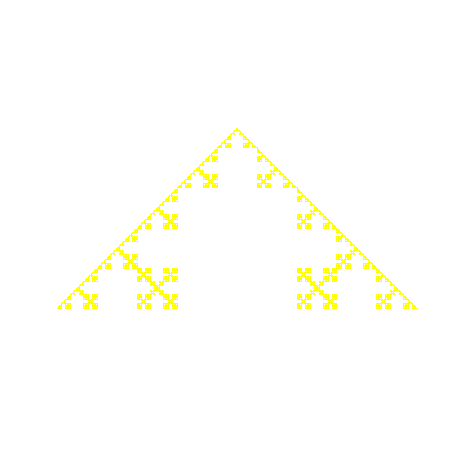30d\$="":mode=1
40 'if d\$="" then input d\$:f=tgcom(d\$):goto 40
50 '
100 f=tgcom("angle 90 move -180 -80 tc 14")
110 f=stitch(360)
120 '
130 func stitch(ln)
140 if ln>4 then
150  f=stitch(ln/3)
160  f=tgcom("left 90")
170  f=stitch(ln/3)
180  f=tgcom("right 90")
190  f=stitch(ln/3)
200  f=tgcom("right 90")
210  f=stitch(ln/3)
220  f=tgcom("left 90")
230  f=stitch(ln/3)
240 else
250  f=tgcom("forward "+str\$(ln))
260 endif
270 endfunc

File [ tg06.bas ]
Load turtle.bas and run, and enter  [ load  tg06.bas ] then drawn.

rp 36 [rp 34 [fd 12 rt 10] rt 90 @1 @1+1 @2 @1%14+1 tc @2]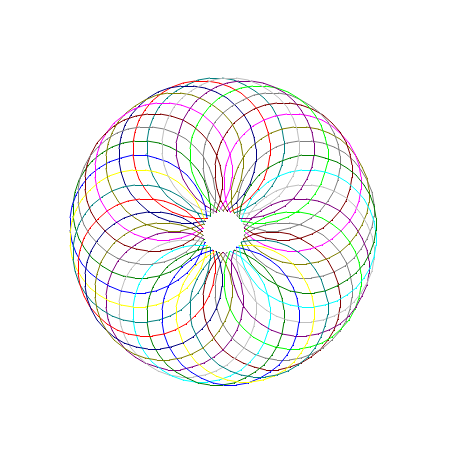[ Back ]